# Chapter 1 First order Partial Differential Equations Sec

• Slides: 9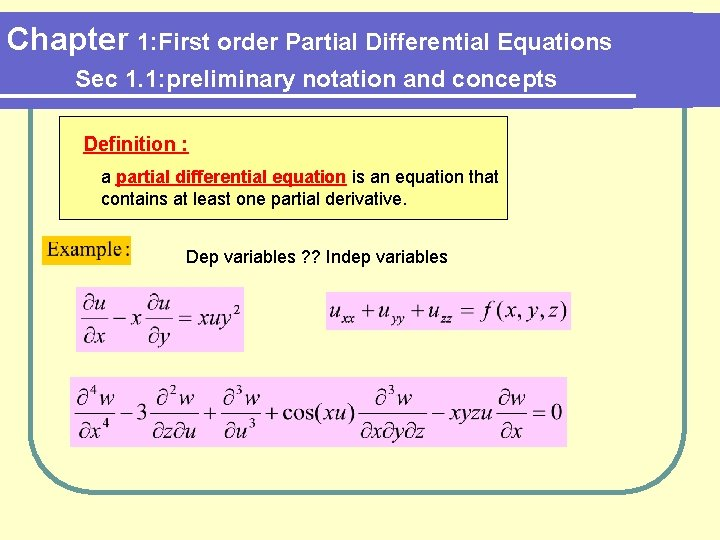Chapter 1: First order Partial Differential Equations Sec 1. 1: preliminary notation and concepts Definition : a partial differential equation is an equation that contains at least one partial derivative. Dep variables ? ? Indep variablesChapter 1: First order Partial Differential Equations Sec 1. 1: preliminary notation and concepts Definition : order of PDE is the order of the highest partial derivative occurring in the equation. Find the order of PDE? ? ?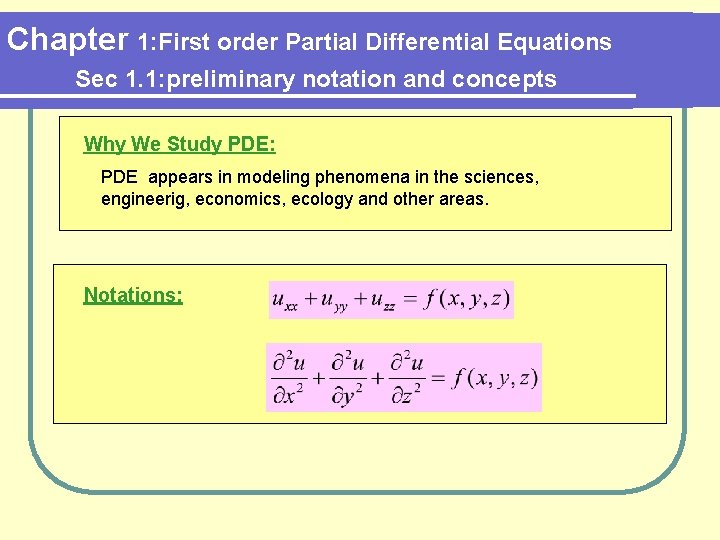Chapter 1: First order Partial Differential Equations Sec 1. 1: preliminary notation and concepts Why We Study PDE: PDE appears in modeling phenomena in the sciences, engineerig, economics, ecology and other areas. Notations: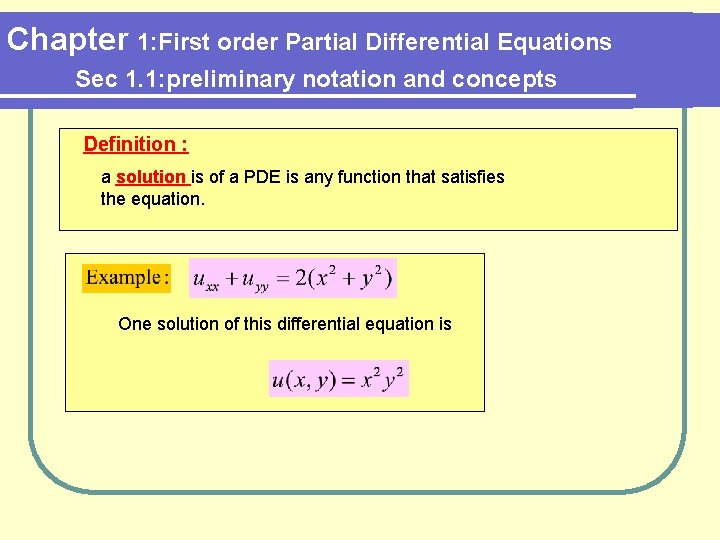Chapter 1: First order Partial Differential Equations Sec 1. 1: preliminary notation and concepts Definition : a solution is of a PDE is any function that satisfies the equation. One solution of this differential equation is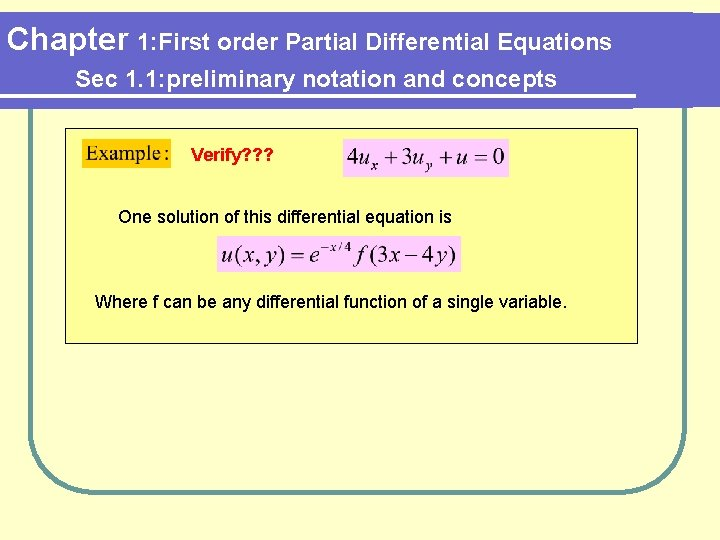Chapter 1: First order Partial Differential Equations Sec 1. 1: preliminary notation and concepts Verify? ? ? One solution of this differential equation is Where f can be any differential function of a single variable.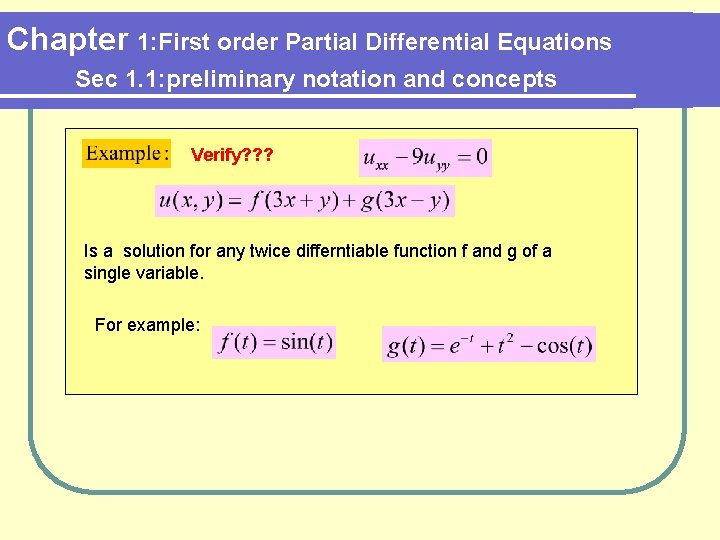Chapter 1: First order Partial Differential Equations Sec 1. 1: preliminary notation and concepts Verify? ? ? Is a solution for any twice differntiable function f and g of a single variable. For example: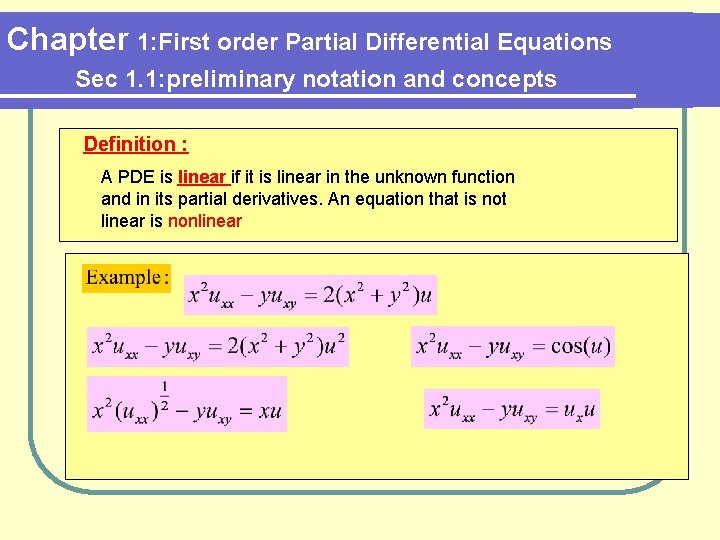Chapter 1: First order Partial Differential Equations Sec 1. 1: preliminary notation and concepts Definition : A PDE is linear if it is linear in the unknown function and in its partial derivatives. An equation that is not linear is nonlinear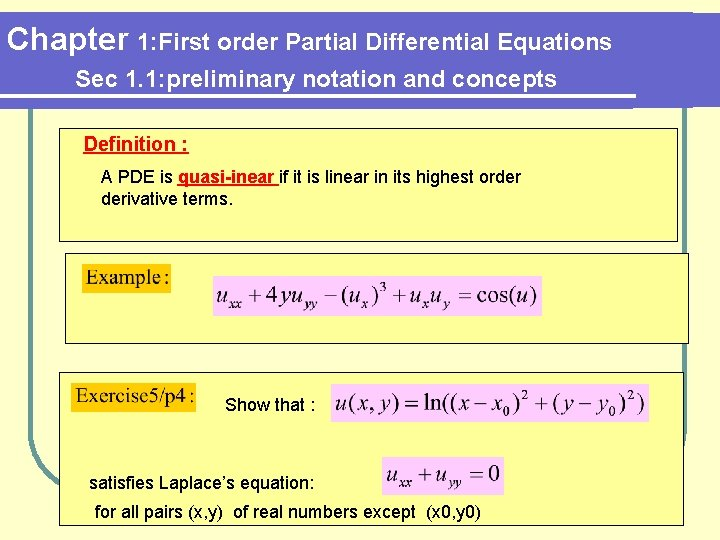Chapter 1: First order Partial Differential Equations Sec 1. 1: preliminary notation and concepts Definition : A PDE is quasi-inear if it is linear in its highest order derivative terms. Show that : satisfies Laplace’s equation: for all pairs (x, y) of real numbers except (x 0, y 0)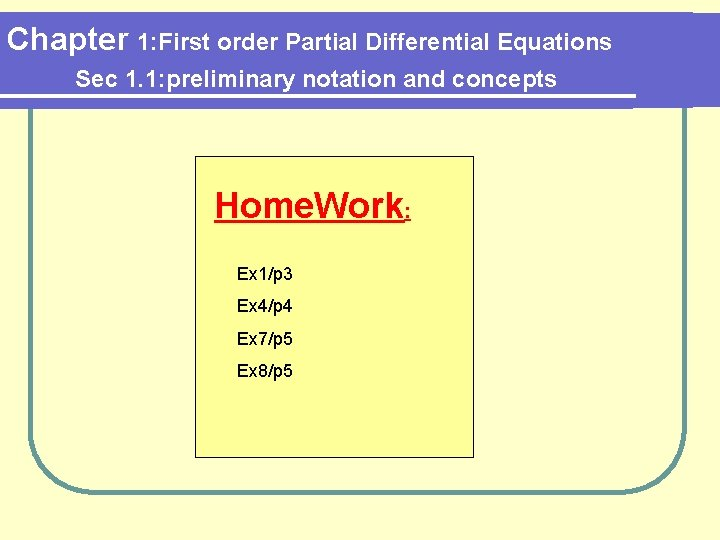Chapter 1: First order Partial Differential Equations Sec 1. 1: preliminary notation and concepts Home. Work: Ex 1/p 3 Ex 4/p 4 Ex 7/p 5 Ex 8/p 5